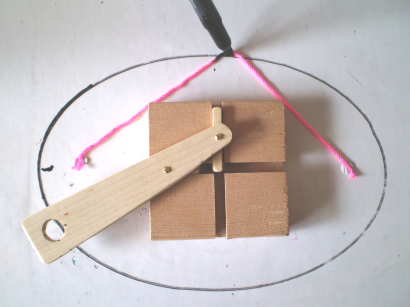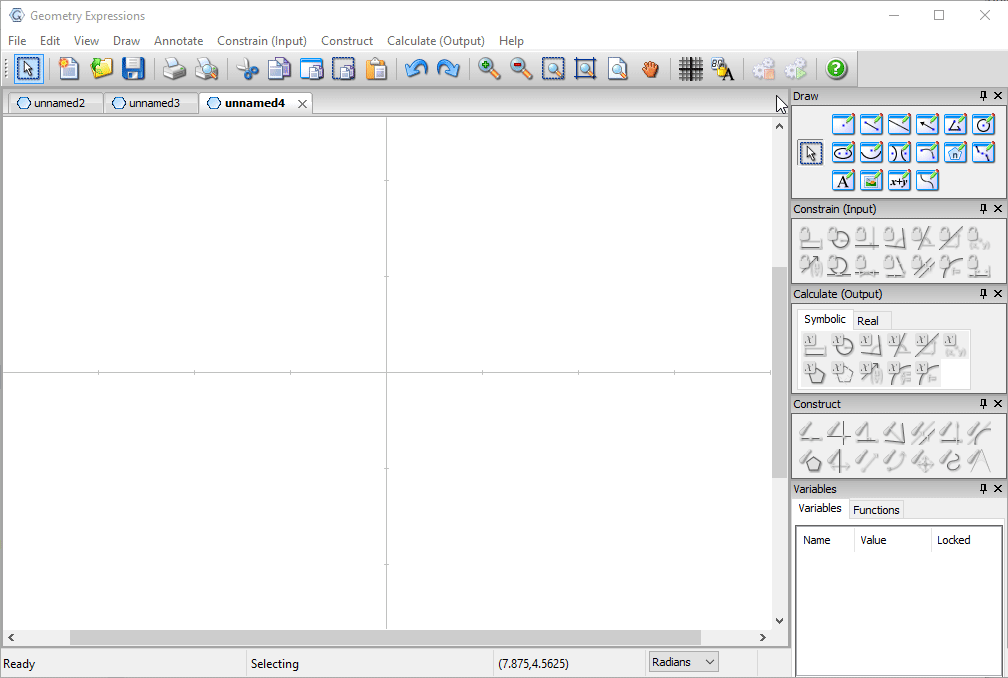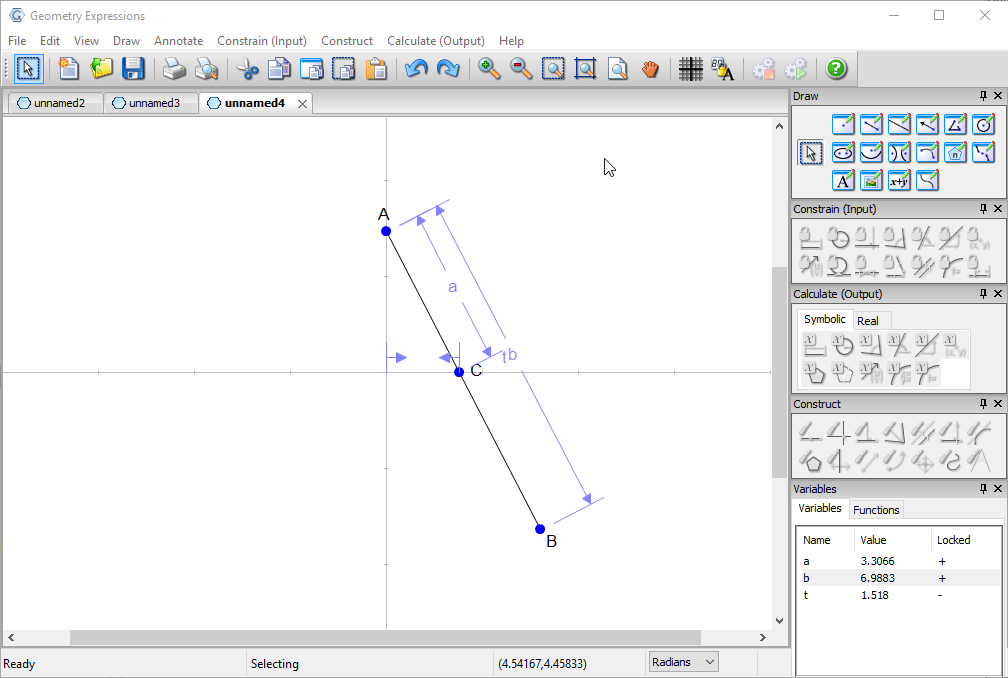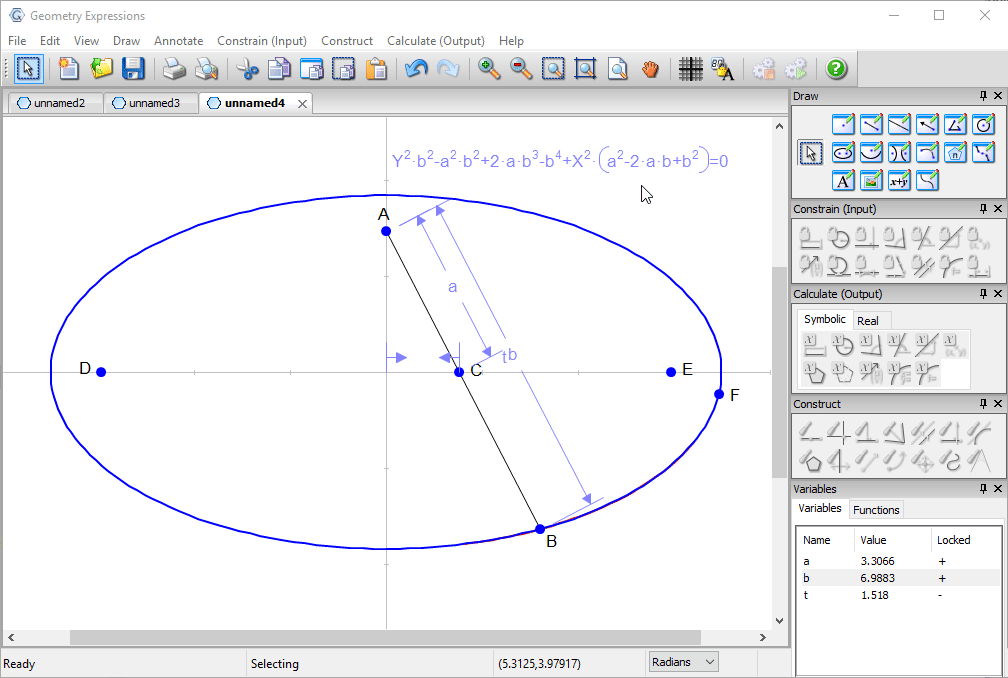# Geometry Expressions## Trammel

Archimedes trammel is a simple device for drawing an ellipse. Another way to draw an ellipse uses a piece of string and two pins.

To match the ellipse drawn by a particular trammel, how long should the string be, and where should the pins go?

We model the trammel as a line segment AB with point A on the y-axis. We place a point C at the intersection of the segment and the x-axis.

We constrain the distances AB and AC and the location (effectively x-coordinate) t of C along the x-axis.We find the locus of point B as t varies.

We measure the equation of the curve and observe that it is quadratic.

We copy the equation for later use.We now draw an ellipse and constrain its equation, pasting in the previously copied curve equation.We can look at the coordinates of the foci, and measure the distance between a focus and a point at the intersection of the ellipse and the y-axis. By symmetry the string will be twice this length.